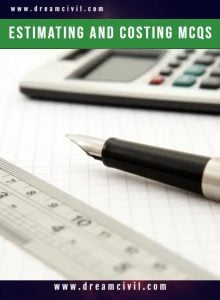Monday, October 2, 2023

Estimating & Costing Objective Questions ( MCQs ) PDF containing 95 questions is given below. You can directly download this PDF and study from it.

### 1. Details of the PDFTopic of MCQs Estimating & Costing Size 240 KB Pages 20 Number of Questions 95

### 2. Some questions from PDF

Question No. 01
The rate of payment is made for 100 cu m (per % cu m) in case of

(A) Earthwork in excavation
(B) Rock cutting
(C) Excavation in trenches for the foundation
(D) All the above

Question No. 02
The rate of an item of work depends on

(A) Specifications of works
(B) Specifications of materials
(C) Proportion of mortar
(D) All the above

Question No. 03
The main factor to be considered while preparing a detailed estimate, is

(A) Quantity of the materials
(B) Availability of materials
(C) Transportation of materials
(D) All the above

Question No. 04
Pick up the correct statement from the following:

(A) The estimated value of the work excluding the amount for contingencies, work charged establishment, tool and plants are called work value
(B) The actual expenditure involved completing work including the incidental, establishment and travelling charges is called actual cost
(C) The formal acceptance by the administrative department for incurring expenditure on the work, is called administrative approval
(D) All the above

Question No. 05
Brick walls are measured in sq. m if the thickness of the wall is

(A) 10 cm
(B) 15 cm
(C) 20 cm
(D) None of these

Question No. 06
The plinth area of a building does not include

(A) Area of the walls at the floor level
(B) Internal shaft for sanitary installations up to 2 sq m. in area
(C) Lift and wall including landing
(D) Area of the cantilevered porch

Question No. 07
If the formation level of a highway has a uniform gradient for a particular length, and the ground is also having a longitudinal slope, the earthwork may be calculated by

(A) Mid-section formula
(B) Trapezoidal formula
(C) Prismoidal formula
(D) All the above

Question No. 08
While estimating a reinforced cement structure, the omitted cover of concrete is assumed

(A) At the end of the reinforcing bar, not less than 25 mm or twice the diameter of the bar

(B) In thin slabs, 12 mm minimum or diameter of the bar whichever is more
(C) For reinforcing longitudinal bar in a beam 25 mm minimum or diameter of the largest bar which is more
(D) All the above

Question No. 09
A cement concrete road is 1000 m long, 8 m wide and 15 cm thick over the sub-base of 10 cm thick gravel. The box cutting in road crust is

(A) 500 m3
(B) 1000 m3
(C) 1500 m3
(D) 2000 m3

Question No. 10
While estimating the qualities for the construction of a building, the correct metric unit is

(A) Metre for length
(B) Cubic metre for the area
(C) Square metres for volume
(D) Litre for capacity

Question No. 11
Pick up the correct statement from the following:

(A) In order to check up the average depth of excavation, ‘Deadman s’ is left at the midwidths of borrow pits

(B) The earthwork calculation in excavation is made from the difference in levels obtained with a level
(C) The earthwork in excavation to form the road embankment includes the formation of correct profile and depositing the soil in layers
(D) All the above

Question No. 12
The brickwork is not measured in cu m in case of

(A) One or more than one brick wall
(B) Brickwork in arches
(C) Reinforced brickwork
(D) Half brick wall

Question No. 13
Pick up the incorrect statement from the following:

(A) Lead is the average horizontal straight distance between the borrow pit and the place of spreading soil
(B) The lead is calculated for each block of the excavated area
(C) The unit of lead is 50 m for a distance up to 500 m
(D) The unit of lead is 1 km where the lead exceeds 2 km

Question No. 14
The assumption on which the trapezoidal formula for volumes is based is

(A) The end sections are parallel planes
(B) The mid-area of a pyramid is half the average area of the ends
(C) The volume of the Prismoidal is over-estimated and hence a Prismoidal correction is applied
(D) All the above

Question No. 15
In the mid-section formula

(A) The mean depth is the average of depths of two consecutive sections
(B) The area of mid-sections is calculated by using mean depth
(C) The volume of the earthwork is calculated by multiplying the mid-section area by the distance between the two original sections
(D) All of the above

Thank you ! for visiting us. If you have any queries then kindly mail us at madhukrishnapoudel151@gmail.com or WhatsApp us at 9804406747.He is a founder and lead author of Dream Civil International and his civil engineering research articles has been taken as source by world's top news and educational sites like USA Today, Time, The richest, Wikipedia, etc.
Latest Articles

Related Articles